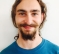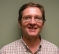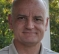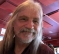## IFFT Synthesis Amplitude EstimationStarted by 3 years ago16 replieslatest reply 3 years ago269 views

Hello there,

I have a simple qustion. I'm working on a IFFT synthesis application - i.e. specify the desired phase and amplitude of each frequency component in order to get a certain waveform in time domain. The question:  is there a way to estimate the amplitude of the output signal if there are more than one frequency components?

The algorithm has to be implemented on FPGA, hence the aplitude has a fixed range (e.g. -1 to 1) however, so far if multiple frequency components are used the resulting aplitude exceeds the desired range. I noticed that the amplitude of the product depends of the amplitude and the phase of each individual bin, therefore I guess there should be some relation between these two parameters and the amplitude of the time domain result

Thanks,                                                                                                                                                    Dimitar

[ - ]As others have noted you will see a peak that is related to the relative phase and power of your input frequency terms.  To see the pathological case put in repeated terms with all the same phase (same value).  That is the spectrum of an impulse and you will get a clipped peak.  It is related to Gibb's phenomena.

Assuming that your input symbols are "whitened" WRT to phase then the output will be more behaved.

A note on scaling.  I struggled with this a bit myself until I realized that the easiest way to look at the problem is in terms of power.  The sum of the power of all the elements in the frequency domain should be equal to the sum of the power of all the elements from the corresponding time sequence.  The difference between the two is accounted for by the scaling factor in the FFT/IFFT.

Another way to look at the problem is to note that other than the scale factor you can implement an IFFT with an FFT.  Take the input values and negate the imaginary terms.  Then take the output values and again negate the imaginary terms.  Compare the results with an IFFT function.

So taking that as a given, think about taking the 256 point FFT of a static signal set to 1.  The output is a DC term of 256 with the rest of the buckets equal to 0.  This is analogous to putting in repeated values in an IFFT and getting an impulse out.  The Peak to Average Power Ratio is not good.

As for the discontinuities between FFT frames they could be improved with the overlap-add method and some tapering.  Just a thought.

Hope some of this helps.

Mark Napier

[ - ]For scaling fft/ifft I choose whatever suits my purpose and it doesn't have to be power unity issue but rather aiming at best dynamic range for a given case.

The power unity issue is purely for math people.

[ - ]This problem is studied extensively in the context of OFDM modulation in data communications, where the power amplifiers are non-linear as you push closer to full power.
Look for documentation on issues of peak-to-average ratio (PAR) for OFDM transmitters.
This is sometimes termed the "crest factor".
In OFDM, a lot of research has been done to play with phase relationships between the bins to try to reduce the PAR.
I'm sure that you will find some interesting techniques being explored.

One question comes to mind: Isn't the ear supposed to be only marginally sensitive to phase (unless you are doing 3D audio)?
If this is true, you can play with the phase to reduce the PAR.

[ - ]I would suggest any one of the following or their combinations, for audio:

1. check the power in FFT domain before taking IFFT. if the power is higher than -3dBFS scale all the band or dominant band(s). The scaling can be slewed. Hope you're taking IFFT with overlap and add (OLA) with windowing. in that case the FFT domain scaling will have only minimum clicks even without any additional slewing.

2. put a dynamic limiter or dynamic processor in time domain. #1 is almost a dynamic limiter in freq domain.

3. Check the time-domain amplitude and keep a feedback to the FFT domain to influence the scaling scheme.

Chalil

[ - ]If the amplitudes and/or phases of the components are random, then they'll reinforce/cancel randomly.   You can bound the extremes by assuming at one point all of the components may add in phase, or cancel, but unless everything is deterministic each instance may be random.

This is why the Peak to Average Power Ratio (PAPR) is problematic for OFDM communication signals, since the subcarriers can add randomly depending on the relative phases.   Same idea.

[ - ]why not just do Wavetable Synthesis?  you can IFFT and scale the waveforms in advance.  is there a reason you must do it real time on the FPGA?

[ - ]The application is a synthesizer and it has wavetable synthesis as an option, however, I want to have additive synthesis capabilities so the designers can craft sounds entirely in frequency domain.

[ - ]Okay, if the waveform is a quasi-periodic note or tone, then all of the "partials" or overtones are virtually harmonic, i.e. every sinusoid (or partial) has frequency that is very nearly an integer multiple of a common fundamental frequency (which is the reciprocal of the period), then all of this sound-crafting in the frequency domain can still be done in advance to the real-time playback and the wavetables are defined from that.

If you want to do additive synthesis in real time (the only real necessity would be if there are multiple partials that are not harmonically related to a common fundamental) then I still would not recommend IFFT to do it.  There are many many issues to worry about:

1. Each sinusoid will have frequency that is likely not exactly equal to an FFT bin frequency.  Then you will have the sinc-like function in the surrounding FFT bins that you must calculate (in real time).

2. Between frames you will have some painful transition stuff to worry about when one frame ends and the next frame begins, if you don't want to hear clicks or "roughness", sorta like zipper noise.

If you're gonna do real-time additive synthesis with possibly non-harmonic partials, then just do it the straight-forward way and add up the separate sinusoids, each with their own phase-accumulator and their own amplitude. Because frequency and phase are strictly coupled (phase is the integral of frequency) you cannot control both independently.  You can independently set the initial phase of each partial, but then you must let the phase fall where it may, given the possibly time-variant frequency of each independent partial.

BTW, this paper is a quarter-century old, and it is sorta academic, but it's about how to extract wavetables from a given sampled note or tone, or how to define the wavetables in terms of the additive synthesis envelopes.  And how to consider the error when reducing data.

Wavetable Synthesis 101, A Fundamental Perspective

[ - ]Thanks a lot for the insigh. I still haven't completely decided on which approach to take so your recommendation is stll an option. If you have some more suggestions of sources you are welcome to throw them in.

[ - ]I can send you some C code that does wavetable synthesis with linear interpolation between samples (this is good enough if the wavetables are large enough that the waveforms are "oversampled") and does linear cross-fading between sequential wavetables or adjacent wavetables along some other parameter axis (in addition to "t") such as pitch or key-velocity or mod-wheel position or slider position.  It doesn't define the wavetables which are assumed defined in advance.  That is from the analysis process of a given note or from the "sound crafting" process.

If the waveforms are sufficiently oversampled and memory is cheap, then I might recommend a decently large power of 2 for the wavetable size.  Like something like 2048 or 4096 points per wavetable.

Feel free to email me at rbj@audioimagination.com and I'll send you a single C source file.  It might show you what you need to get your FPGA to do on the sample-processing level.  Computing what the wavetables will need to be is the big hard problem (but not too hard, that's what the paper is about).

[ - ]You do not aim for a specific waveform of the output. Since your requirement is that the amplitude of the sum of the sinusoids not exceed a specific range, say +-A, wouldn't be simpler to simply divide by the max output sum amplitude, and then multiply by A?

[ - ]It is an opion, but I thought there may be other ways.

[ - ]Since your platform is fpga I expect you will use fft core from a vendor. I also assume you are using fixed pont processing. vendor fft cores use block floating point to care for internal bit growth and output a scale factor... thus the matlab fft will not reflect what you get from core and you better have model of vendor core instead.

[ - ]Floating point is also an option, but I'll have to do some more research in on this topic. Thanks for the suggestion though.

[ - ]